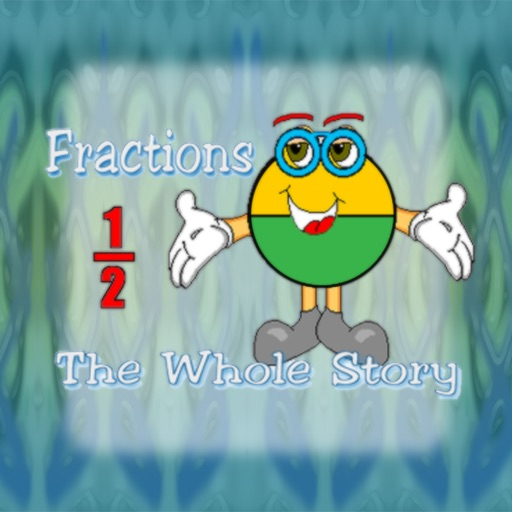Fractions: The Whole Story# Fractions: The Whole Story

## Ventura Educational Systems

2.0 for iPhone, iPad
\$0.99
0

0 Ratings

Release Date

2016-11-09

Size

49.0 MB

### Screenshots for iPhone

iPhone
More Screenshots for iPad
Fractions: The Whole Story Description
In the era of computers and calculators, why is it important for students to learn fractions? A student must understand fractions in order to learn algebra and geometry. In addition to recognizing the parts of a fraction, young learners need to understand the meaning of each part and its relationship to the whole. Because many young learners have difficulty developing the concept of fractions, using pictures of shapes and groups of objects to represent fractions enables young learners to understand their meaning. Then, students can make the transition from the pictorial representation of fractions to abstract mathematical notation. This understanding allows them to move on to more advanced mathematics.

First, it is important for the child to understand that traditional representations of fractions have three parts: the numerator, the fraction bar and the denominator. Proper fractions are used to represent parts of a whole. The denominator tells into how many equal parts the whole is divided. The numerator tells how many of those parts are being considered. The fraction bar is a symbol for division.

Usually basic fractions concepts are represented in one of two ways; either by a shape divided into equal parts with some of the parts shaded, or by presenting a group of objects with some of the objects different in some way. Children should recognize both representations for fractions. For example, dividing a circle into four equal parts is one way to show the fraction one-fourth. Another way is to have a group of four objects with one or more of the objects different in some way.

Strong multiplication skills are a prerequisite to understanding and using fractions. In order to use Least Common Multiple (LCM) to compare and add fractions students must know the multiples of two numbers. LCM is used for finding equivalent fractions with common denominators.

How can you help a young learner understand fractions? Talk about the meaning of fractions by pointing out examples in your environment. How many cars in a row of the parking lot? What fraction are white cars? Point out how fractions are used when following a recipe or measuring wood for a building project. Practice multiplication by counting aloud together by two’s, three’s, and four’s, etc. Compare two sets of multiples (for example, 4,8,12,16, 20, 24... and 5, 10, 15, 20, 25...) and let your child discover the first number that appears in both sets. This number is the LCM. You’ll be amazed at how much a student will learn just by talking about fractions in everyday life.

How quickly students learn to work with fractions depends on many factors. But once they have mastered fractions, they will be ready to take on the challenges of higher math, especially algebra and geometry.

Fractions: The Whole Story is a fun, convenient way to introduce and reinforce basic fraction concepts. For all the times you and your student have a minute or two, this iPhone application is the perfect opportunity to practice multiplication skills. It will entertain both of you while you’re waiting in line at the grocery store, sitting in the waiting room at the dentist, or traveling by bus or train. The possibilities are endless!
Fractions: The Whole Story 2.0 Update
2016-11-09 Version History
This version provides support for iOS 10 and higher.
More